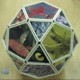# Polymorphous Pursuit

Suppose four mice are standing on the corners of a large square. Each mouse begins running at the same speed towards the mouse one place away, reckoning clockwise. The mice will meet at the centre of the square and the path taken by each mouse will be what is known as a pursuit curve:vertices = 4, mouse-increment = 1v = 4, mi = 1 (animated)

As I showed in “Persecution Complex”, it’s easy to find variants on the basic pursuit curve. If mi = 2, i.e. each mouse runs towards the mouse two places away, the mice will run in straight lines direct to the centre of the square:v = 4, mi = 2v = 4, mi = 2 (animated)

That variant is trivial, but suppose there are eight mice, four starting on the corners of the square and four starting on the midpoints of the sides. Mice starting on the corners will run different pursuit curves to those starting on the midpoints, because the corners are further from the centre than the midpoints are:v = 4, si = 1, mi = 1If mi = 3, the pursuit curves look like this:v = 4, si = 1, mi = 3v = 4, si = 1, mi = 3 (animated)

Suppose there are twelve mice, four on each corner and two more on each side. If each mouse runs towards the mouse four places away, then the pursuit curves don’t all meet in the centre of the square. Instead, they meet in groups of three at four points equidistant from the centre, like this:v = 4, si = 2, mi = 4v = 4, si = 2, mi = 4 (animated)v = 4, si = 4, mi = 4 (animated)v = 4, si = 4, mi = 4 (zoom)

Now suppose each mouse become sophisticated and runs toward the combined positions of two other mice, one two places away, the other three places away, like this:v = 4, si = 1, mi = (2, 3)v = 4, si = 1, mi = (2, 3) (animated)

These polypursuits, as they could be called, can have complicated central regions:v = 4, si = 2, mi = (1, 4)v = 4, si = 2, mi = (1, 4) (animated)v = 4, si = various, mi = various

And what if you have two teams of mice, running towards one or more mice on the other team? For example, suppose two mice, one from each team, start on each corner of a square. Each mouse on team 1 runs towards the mouse on team 2 that is one place away, while each mouse on team 2 runs towards the mouse on team 1 that is two places away. If the pursuits curves of team 1 are represented in white and the pursuit curves of team 2 in green, the curves look like this:v = 4 * 2, vmi = 1, vmi = 2v = 4 * 2, vmi = 1, vmi = 2v = 4 * 2, vmi = 1, vmi = 2 (animated)

Now suppose the four mice of team 1 start on the corners while the mice of team 2 start at the centre of the square.v = 4, centre = 4, vmi = 1, cmi = 2 (white team)v = 4, centre = 4, vmi = 1, cmi = 2 (green team)v = 4, centre = 4, vmi = 1, cmi = 2 (both teams)v = 4, centre = 4, vmi = 1, cmi = 2 (animated)

Here are more variants on pursuit curves formed by two teams of mice, one starting on the corners, one at the centre:v = 4, centre = 4, vmi = (0, 1), cmi = 0v = 4, centre = 4, vmi = (0, 2), cmi = 0v = 4, centre = 4, vmi = (0, 3), cmi = 0## 2 thoughts on “Polymorphous Pursuit”

1.MawBTS says:

Do you think you could make a perpetual motion machine? (A configuration of mice that chase each other forever and never meet?)

It would probably be relatively easy if the mice had variable behavior (under certain conditions they chase the n+1 mouse, while under other conditions they chase the n+2 mouse, or something).

•Krilling for Company says:

Interesting question. If there were two or more sets of mice, you could set up a kind of bouncing movement by e.g. making all even mice in set 1 chase all even (or odd) mice in set 2, while all odd mice in set 2 starting chasing all odd (or even) mice in set 1. But I don’t think you could do by adjusting variables in a single set of mice.

If the mice are regarded as infinitesimal points all following standard pursuit curves, then I assume that they only meet after an infinitely long time if they move at constant speed.

UPDATE: I’m talking rubbish: they obviously meet in finite time, although they pass through an infinite number of points to get to the centre. See Zeno’s paradoxes.

This site uses Akismet to reduce spam. Learn how your comment data is processed.